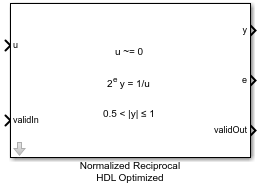# Normalized Reciprocal HDL Optimized

Computes normalized reciprocal and generates optimized HDL code

• Library:
• Fixed-Point Designer HDL Support / Math Operations

•## Description

The Normalized Reciprocal HDL Optimized block computes the normalized reciprocal of u, returned as y and t such that 0.5 < |y| ≤ 1 and 2ey = 1/u.

• If u = 0 and u is a fixed-point or scaled-double data type, then y = 2 – eps(y) and e = 2nextpow2(w)w + f, where `w` is the word length of u and f is the fraction length of u.

• If u = 0 and u is a floating-point data type, then y = `Inf` and t = 1.

## Ports

### Input

expand all

Value to take the normalized reciprocal of, specified as a real scalar.

Data Types: `single` | `double` | `fixed point`

Whether input is valid, specified as a Boolean scalar. This control signal indicates when the data from the u input port is valid. When this value is `1` (`true`), the block captures the value at the u input port. When this value is `0` (`false`), the block ignores the input samples.

Data Types: `Boolean`

### Output

expand all

Normalized reciprocal that satisfies 0.5 < |y| ≤ 1 and 2ey = 1/u, returned as a scalar.

• If the input at port u is a signed fixed-point or scaled-double data type with word length w, then y is a signed fixed-point or scaled-double data type with word length w and fraction length w – 2.

• If the input at port u is an unsigned fixed-point or scaled-double data type with word length w, then y is an unsigned fixed-point or scaled-double data type with word length w and fraction length w – 1.

• If the input at port u is a double, then y is a double.

• If the input at port u is a single, the y is a single.

Data Types: `single` | `double` | `fixed point`

Exponent that satisfies 0.5 < |y| ≤ 1 and 2ey = 1/u, returned as an integer scalar.

Data Types: `int32`

Whether the output data is valid, returned as a Boolean scalar. When the value of this control signal is `1` (`true`), the block has successfully computed the outputs at ports y and e. When this value is `0` (`false`), the output data is not valid.

Data Types: `Boolean`

## Algorithms

The Normalized Reciprocal HDL Optimized block works by normalizing the input using a binary search, which has a latency of approximately log2 of the word length of the input, followed by a CORDIC reciprocal kernel, which has a latency approximately the same as the word length of the input.

The Normalized Reciprocal HDL Optimized block is always ready to accept data. After the initial latency, valid samples are output every sample. The latency in samples for a fixed-point input u is

` D = ceil(log2(u.WordLength)) + u.WordLength + 5`

## Extended Capabilities

### Blocks

Introduced in R2020a

## SupportGet trial now